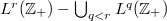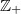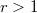﻿

### A spaceability result in the context of hypergroups

#### Abstract

In this paper, by an elementary constractive technique, it is shown that$L^r(\mathbb{Z}_+)-\bigcup_{q is non-empty, where$\mathbb{Z}_+$ is the dual of a compact countable hypergroup introduced by Dunkl and Ramirez. Also, we prove that for each$r>1$,$L^r(\mathbb{Z}_+)-\bigcup_{q is spaceable.

DOI Code: 10.1285/i15900932v38n1p17

Keywords: locally compact hypergroup; spaceability; \$L^p\$-space

Full Text: PDF